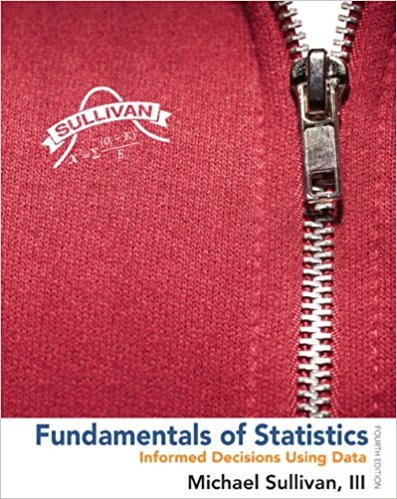×
×

# Solutions for Chapter Chapter 3.2: Fundamentals of Statistics 4th Edition## Full solutions for Fundamentals of Statistics | 4th Edition

ISBN: 9780321838704Solutions for Chapter Chapter 3.2

Solutions for Chapter Chapter 3.2
4 5 0 255 Reviews
17
2
##### ISBN: 9780321838704

Chapter Chapter 3.2 includes 57 full step-by-step solutions. This textbook survival guide was created for the textbook: Fundamentals of Statistics, edition: 4. This expansive textbook survival guide covers the following chapters and their solutions. Since 57 problems in chapter Chapter 3.2 have been answered, more than 288683 students have viewed full step-by-step solutions from this chapter. Fundamentals of Statistics was written by and is associated to the ISBN: 9780321838704.

Key Statistics Terms and definitions covered in this textbook
• Analysis of variance (ANOVA)

A method of decomposing the total variability in a set of observations, as measured by the sum of the squares of these observations from their average, into component sums of squares that are associated with speciic deined sources of variation

• Attribute control chart

Any control chart for a discrete random variable. See Variables control chart.

• Average

See Arithmetic mean.

• Bayes’ estimator

An estimator for a parameter obtained from a Bayesian method that uses a prior distribution for the parameter along with the conditional distribution of the data given the parameter to obtain the posterior distribution of the parameter. The estimator is obtained from the posterior distribution.

• Bivariate normal distribution

The joint distribution of two normal random variables

• Box plot (or box and whisker plot)

A graphical display of data in which the box contains the middle 50% of the data (the interquartile range) with the median dividing it, and the whiskers extend to the smallest and largest values (or some deined lower and upper limits).

• Chance cause

The portion of the variability in a set of observations that is due to only random forces and which cannot be traced to speciic sources, such as operators, materials, or equipment. Also called a common cause.

• Chi-square (or chi-squared) random variable

A continuous random variable that results from the sum of squares of independent standard normal random variables. It is a special case of a gamma random variable.

• Comparative experiment

An experiment in which the treatments (experimental conditions) that are to be studied are included in the experiment. The data from the experiment are used to evaluate the treatments.

• Conditional variance.

The variance of the conditional probability distribution of a random variable.

• Continuity correction.

A correction factor used to improve the approximation to binomial probabilities from a normal distribution.

• Control chart

A graphical display used to monitor a process. It usually consists of a horizontal center line corresponding to the in-control value of the parameter that is being monitored and lower and upper control limits. The control limits are determined by statistical criteria and are not arbitrary, nor are they related to speciication limits. If sample points fall within the control limits, the process is said to be in-control, or free from assignable causes. Points beyond the control limits indicate an out-of-control process; that is, assignable causes are likely present. This signals the need to ind and remove the assignable causes.

• Cumulative normal distribution function

The cumulative distribution of the standard normal distribution, often denoted as ?( ) x and tabulated in Appendix Table II.

• Decision interval

A parameter in a tabular CUSUM algorithm that is determined from a trade-off between false alarms and the detection of assignable causes.

• Enumerative study

A study in which a sample from a population is used to make inference to the population. See Analytic study

• Error variance

The variance of an error term or component in a model.

• Factorial experiment

A type of experimental design in which every level of one factor is tested in combination with every level of another factor. In general, in a factorial experiment, all possible combinations of factor levels are tested.

• Fraction defective

In statistical quality control, that portion of a number of units or the output of a process that is defective.

• Fractional factorial experiment

A type of factorial experiment in which not all possible treatment combinations are run. This is usually done to reduce the size of an experiment with several factors.

• Frequency distribution

An arrangement of the frequencies of observations in a sample or population according to the values that the observations take on

×